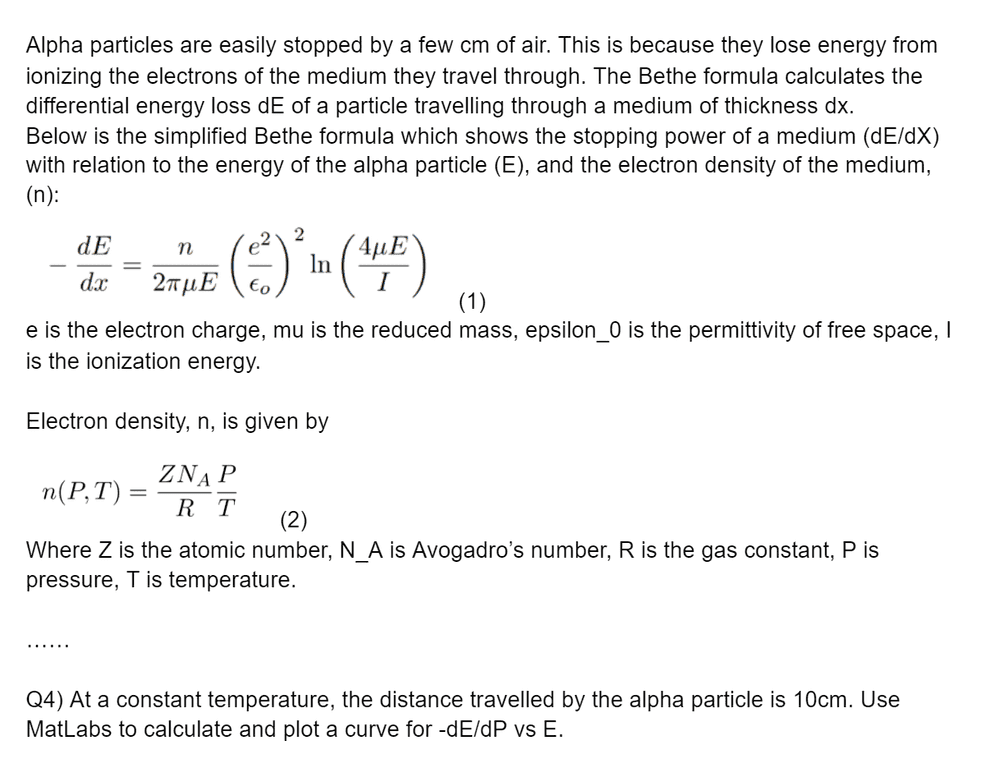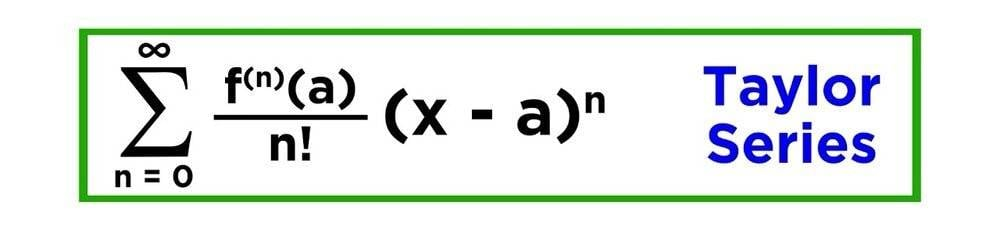# Using chain rule when one of the variables is constant

• PandaKitten
PandaKitten
Homework Statement
-dE/dx = A*(n/E )*ln(E)
n=P/T
Find dE/dP
Where T and A and dx are constants. E and P are variables
Relevant Equations
-dE/dx = A*(n/E )*ln(E)
n=P/T
So first thing I tried was to separate the variables then differentiate by parts, setting u = E and v = 1/ln(E) (and the other way around) but I couldn't do the integral it gave.
Then I tried to reason that because dx was constants then dE/dx is equal to E/x but I was told that's not the case. The lecturer also mentioned the truncated series for a Taylor expansion but I'm not exactly sure how that is relevant

Staff Emeritus
Gold Member
I'm confused how the thing on the right relates to x. What does it mean for dx to be a constant?

In general if you have ##dE/dx=C## for some constant C, then integrating gives you ##E=Cx+D## for an arbitrary constant ##D##. I can't tell if you're trying to say that's the situation you're in.

PandaKitten
The first equation works out the rate of decrease of energy with distance -dE/dx of an energy emitter. But in this question, we are at a fixed distance from the energy emitter. So it wants us to write it in terms of dE/dP instead where P is pressure (second equation). Let me know if it is still unclear. Sorry if this doesn't make sense

PandaKitten
We're at a fixed distance from the emitter so dx is constant**

Gold Member
Would you provide the problem as it was assigned?

PandaKitten
The question sheet includes a lot of background information and other questions. Below is a summary.willem2
You'll need to solve a differential equation for E and x to compute E at x = 10.
You might need a numerical method for this. The Taylor expansion could also be used here (if the error is small enough). This E will of course depend on P, so you can calculate dE/dP. This might have to be done numerically also.

Gold Member
Be careful, when E gets small enough, the ln changes sign, so there is something else that has to be brought in.

PandaKitten
You'll need to solve a differential equation for E and x to compute E at x = 10.
You might need a numerical method for this. The Taylor expansion could also be used here (if the error is small enough). This E will of course depend on P, so you can calculate dE/dP. This might have to be done numerically also.
How would I solve it using the Taylor expansion? Would I use this formula and set X= 10cm?